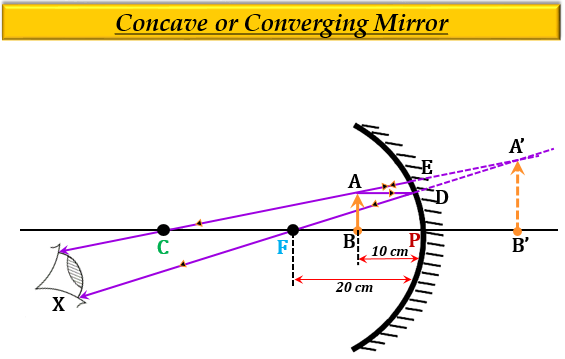# An object is placed at a distance of 10 cm from a concave mirror of focal length 20 cm.(a) Draw a ray diagram for the formation of image.(b) Calculate the image distance.(c) State two characteristics of the image formed.

(a) Ray Diagram-(b) Given:

Distance of the object from the mirror $u$ = $-$10 cm

Focal length of the mirror, $f$ = $-$20 cm

To find: Distance of the image $v$.

Solution:

From the mirror formula, we know that-

$\frac{1}{f}=\frac{1}{v}+\frac{1}{u}$

Substituting the given values in the mirror formula we get-

$\frac{1}{(-20)}=\frac{1}{v}+\frac{1}{(-10)}$

$-\frac{1}{20}=\frac{1}{v}-\frac{1}{10}$

$\frac{1}{10}-\frac{1}{20}=\frac{1}{v}$

$\frac{1}{v}=\frac{2-1}{20}$

$\frac{1}{v}=\frac{1}{20}$

$v=+20cm$

Thus, the distance of the image $v$ is 20cm, and the positive sign implies that the image forms behind the mirror (on the right).

(c) The two characteristics of the image formed are as follows:

1. It is virtual and erect.

2. It is magnified (enlarged).

Updated on: 10-Oct-2022

420 Views